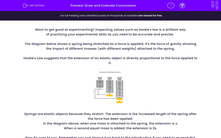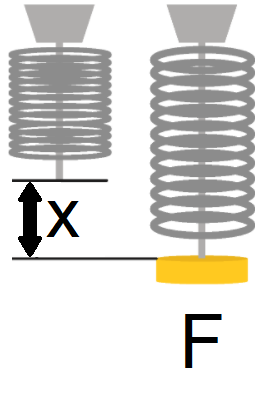# Draw and Evaluate Conclusions

In this worksheet, students will learn about Hooke's law and the impact of a force on a spring. They will then practise drawing conclusions and evaluating their results.Key stage:  KS 3

Curriculum topic:   Working Scientifically

Curriculum subtopic:   Analysis and Evaluation

Difficulty level:#### Worksheet Overview

Want to get good at experimenting? In this activity, we'll inspect values from Hooke's law as a way of practising your experimental skills, to be accurate and precise.

The diagram below shows an unstretched spring with no force applied. If masses are then added, gravity allows the masses to produce a force downwards to stretch the spring. (The force is weight, which is equal to mass x gravity).Let's add a mass onto the spring. It will produce a force, F, and cause an extension of x.When a second equal mass is added, the force increases to 2F and the extension now stretches to 2x.Hooke's Law shows us that the extension of an elastic object is directly proportional to the force applied to it. As we can see, we doubled the force, so the extension doubled.

Springs are elastic objects because they stretch. The extension is the increased length of the spring after the force has been applied. (In other words, extension is the new length of the spring, minus its original length).

Now it's over to you. Have a go at analysing some data from a Hooke's Law experiment.

Remember you can always turn back to the introduction if you need to re-read this information.

### What is EdPlace?

We're your National Curriculum aligned online education content provider helping each child succeed in English, maths and science from year 1 to GCSE. With an EdPlace account you’ll be able to track and measure progress, helping each child achieve their best. We build confidence and attainment by personalising each child’s learning at a level that suits them.

Get started